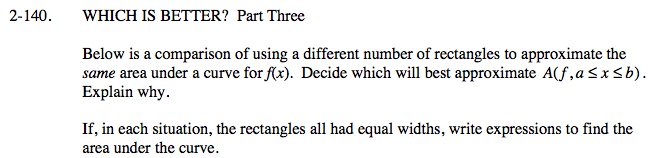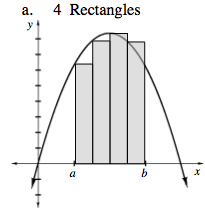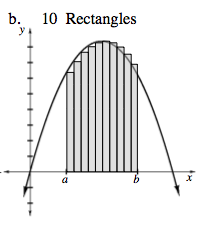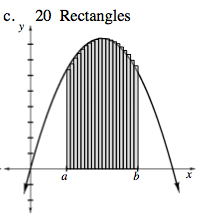### Home > CALC > Chapter Ch2 > Lesson 2.4.1 > Problem2-140

2-140.Look at the shaded area beneath each curve. Which sketch most accurately represents the actual area under the curve?

General form for left-endpoint Riemann sum using sigma notation:$\sum_{i=0}^{3}\frac{b-a}{4}f\left ( a+\frac{b-a}{4}i \right )$$\sum_{i=0}^{9}\frac{b-a}{10}f\left ( a+\frac{b-a}{10}i \right )$$\sum_{i=0}^{19}\frac{b-a}{20}f\left ( a+\frac{b-a}{20}i \right )$